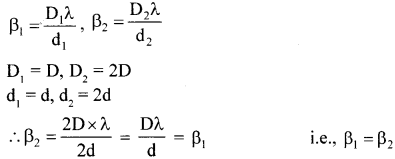# GSEB Solutions Class 12 Physics Chapter 10 Wave Optics

Gujarat Board GSEB Textbook Solutions Class 12 Physics Chapter 10 Wave Optics Textbook Questions and Answers, Additional Important Questions, Notes Pdf.

## Gujarat Board Textbook Solutions Class 12 Physics Chapter 10 Wave Optics

### GSEB Class 12 Physics Wave Optics Text Book Questions and Answers

Question 1.
Monochromatic light of wavelength 589 nm is incident from air on a water surface. What are the wavelength, frequency and speed of (a) reflected and (b) refracted light? Refractive index of water is 1.33.
(a) λ = 589 x 109m,c = 3 x 108m/s (air)
∴Frequency, υ = $$\frac {c}{λ}$$ = $$\frac{3 \times 10^{8}}{589 \times 10^{-9}}$$ = 5.09 x 1014Hz
No change for λ and c

(b) Frequency remains the sanie.
Wave length. λ = $$\frac {λ}{n}$$ = $$\frac {589 nm}{1.33}$$ = 443 nm
Speed, V’ = $$\frac {c}{n}$$ = $$\frac{3 \times 10^{8}}{1.33}$$ = 2.26 x 108 m/s

Question 2.
What is the shape of the wavefront in each of the following cases?
(a) Light diverging from a point source.
(b) Light emerging out of a convex lens when a point source is placed at its focus.
(c) The portion of the wavefront of light from a distant star intercepted by the Earth.
Solution:
(a) Spherical
(b) Plane
(c) Plane

Question 3.
(a) The refractive index of glass is 1.5. What is the speed of light in glass? (Speed of light in ‘vacuunm is 3.0 x 108ms-1)
(b) Is the Speed of light in glass independent of the colour of light? If not, which of the two colours red and violet travels slower in a glass prism?
(a) n = 1.5, c = 3 x 108 m/s
(b) No. Speed depends on colour of light (nv > nR)
Speed of violet component is less than that of red component.Question 4.
In a Young’s double-slit experiment, the slits are separated by 0.28 mm and the screen is placed 1.4 m away. The distance between the central bright fringe and the fourth bright fringe is measured to be 1.2 cm. Determine the wavelength of light used in the experiment.
Solution:
d = 0.28 mm = 0.28 x 10-3m, D = 1.4 m
n = 4. yn = 1.2 x 10-2n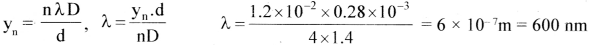Question 5.
In Young’s double-slit experiment using monochromatic light of wave length λ, the intensity of tight at a point on the screen where path difference is λ, is K units. What is the intensity of light at a point where path difference is $$\frac {λ}{3}$$?
Solution:
Interference of waves from the two slits gives:
Intensity, I = I1 + I2 + 2$$\sqrt{\mathrm{I}_{1} \mathrm{I}_{2}}$$. cos ∅
Phase difference. ∅ = $$\frac {2π}{λ}$$ x path difference = $$\frac {2π}{λ}$$ x λ= 2π =0°
∴ Intensity. I = I + I + 2$$\sqrt{1 . I}$$. cos 0 = I + I + 2I = 41 = K units (given)
For path difference $$\frac {λ}{3}$$,∅ = $$\frac {2π}{λ}$$ x $$\frac {λ}{3}$$ = $$\frac {2π}{3}$$ = 120°
∴ Intensity, I’ = I + I + 2$$\sqrt{1 . I}$$ cos 120 = I + I + 2I$$\left(\frac{1}{-2}\right)$$ = I = $$\frac {K}{4}$$ (unit)

Question 6.
A beam of light consisting of two wavelengths, 650 n.u and 520 urn, is used to obtain interference fringes in a Young’s double-slit experiment.
(a) Find the distance of the third bright fringe on the screen from the central maxi mum for wavelength 650 nm.
(b) What is the least distance from the central maximum where the bnglit fringes due to both the wavelengths coincide?
Solution:
(a) λ = 650 x 10-9m, d =2 x 10-3m, D = 1.2m,n = 3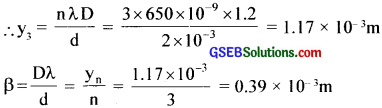(b)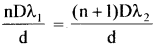(higher order of lower wavelength coincides with lower order of higher wavelength)
n λ1 = (n + 1)λ1, $$\frac{n}{n+1}$$ = $$\frac{\lambda_{2}}{\lambda_{1}}$$ = $$\frac{520}{650}$$ = 0.8
n = 0.8 n + 0.8, 0.2n = 0.8 ∴ n = 4
λn = $$\frac{\mathrm{nD} \lambda_{1}}{\mathrm{~d}}$$ = nβ = 4 x 39 x 10-3 mQuestion 7.
In a double-slit experiment the angular width of a fringe is found to be 0.2° on a screen placed 1 m away. The wavelength of light used is 600 nm. What will be the angular width of the fringe if the entire experimental apparatus is immersed in water? Take refractive index of water to be $$\frac{4}{3}$$
Solution:
Angular size θ = $$\frac{λ}{d}$$is independent of D.
∴ $$\frac{\theta^{\prime}}{\theta}=\frac{\lambda^{\prime}}{\lambda}$$ But λ’ = $$\frac{λ}{n}$$
where ‘n’ is the refractive index.
∴ θ’ = $$\frac{\theta}{\lambda^{\prime}} \times \frac{\lambda^{\prime}}{n}=\frac{\theta}{n}=\frac{0.2^{\circ}}{(4 / 3)}$$ = 0.15°

Question 8.
What is the Brewster angle for air to glass transition? (Refractive index of glass = 1.5)
Solution:
n tan ip ∴ ip = tan-1n = tan-1(1.5) = 56.3°

Question 9.
Light of wavelength 5000 Å falls on a plane reflecting surface. What are the wave length and frequency of the reflected light? For what angle of incidence is the reflected ray normal to the incident ray?
Solution:
λ = 5 x 10-7m, c = 3 x 108ms-1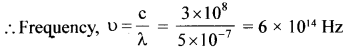i = r, i + r = 90° ∴ 2i = 90°, i = 45°

Question 10.
Estimate the distance for which ray optics is good approximation for an aperture of 4 mm and wavelength 400 nm.
Expression for Fresnel distance is: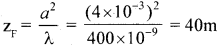Question 11.
The 6563 A Ha line emitted by hydrogen in a star is found to be redshifted by 15 A. Estimate the speed with which the star is receding from the Earth.
Solution:
λ = 6563 x 10°m. ∆λ = 15 x 10-10m. λ’ = λ + ∆λ = 6578 x 10-10m
λ’ – λ = $$\frac{-v}{c}$$λ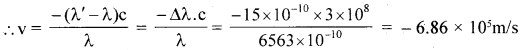Question 12.
Explain how Corpuscular theory predicts the speed of light in a medium, say, water, to be greater than the speed of light in vacuum. Is the prediction confirmed by experimental determination of the speed of light in water? If not, which alternative picture of light is consistent with experiment?
Solution:
In corpuscular theory refraction is due to attraction of light particles and that of refracting medium. This results in an increase of the velocity. This means
c sin i = v sin r or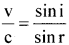= n. Since n> 1, v > c
The prediction is opposite to the experimental results (v <c). The wave picture of light is consistent with the experiment.Question 13.
You have learnt ¡n the text how Huygens’ principle leads to the laws of reflection and refraction. Use the same principle to deduce directly that a point object placed in front of a plane mirror produces a virtual ¡mage whose distance from the mirror is equal to the object distance from the mirror.
Solution:
From the object spherical wave fronts reach the reflector. Then reflected wave fronts are obtained from the mirror. One such wavefront that develops from the mirror in time ‘t’ seconds is shown as reflected wavefront. If un- reflected, in the absence of mirror, ADB is the position in the forward direction. Chord AB being the same, the two arcs ADB and ACB should have their centres equidistant. i.e., ON = Nl i.e., the image is formed as far behind the mirror as the object is in front of it.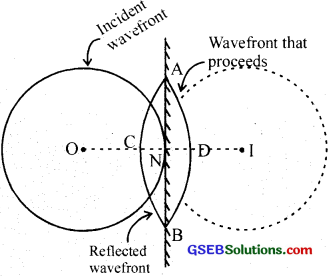Question 14.
Let us list some of the factors, which could possibly influence the speed of wave propagation.
i. Nature of the source.
ii. Direction of propagation.
iii. Motion of the source and/or observer.
iv. Wavelength.
v. Intensity of the wave.
On which of these factors, if any, Does
(a) the speed of light in vacuum,
(b) the speed of light in a medium (say, glass or water), depend?
(a) h is a fundamental constant. Independent of relative motion of source and observer.
(b) Does not depend on the nature of the source, direction of propagation (in the case of homogeneous isotropic medium), motion of the source and intensity (except for very’ high frequencies). It is seen that velocity depends on wavelength and the nature of the medium.Question 15.
For sound waves, the Doppler formula for frequency shift differs slightly between the two situations: (i) source at rest; observer moving, and (ii) source moving; observer at rest. The exact Doppler formulas for the case of light waves in vacuum are, however, strictly identical for these situations. Explain why this should be so. Would you expect the formulas to be strictly identical for the two situations in case of light travelling in a medium?
Solution:
Sound requires a material medium for its propagation. The relative motion of source and listener, need not be identical. In vacuum, light has the same speed irrespective of motion of source and observer – hence results of Doppler effect are identical. But in a medium, light produces Doppler effect similar to sound.

Question 16.
In double-slit experiment using light of wavelength 600 nm, the angular width of a fringe formed on a distant screen is 0.1°. What is the spacing between the two slits?
Solution:
d sin θ = n λ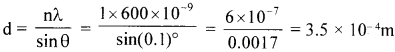Question 17.
(a) In a single slit diffraction experiment. the width of the slit is made double the original width. How does this affect the sue and intensity of the central diffraction band?

(b) In what way is diffraction from each slit related to the interference pattern in a double-slit experiment?

(c) When a tiny circular obstacle is placed in the path of light from a distant source, a bright spot is seen at the centre of the shadow of the obstacle. Explain why.

(d) Two students are separated by a 7 m partition wall in a room 10 m high. if both light and sound waves can bend around obstacles, how are the students unable to see each other even though they can converse easily.

(d) Ray optics is based on the assumption that light travels in a straight line. Diffraction effects (observed when light propagates through small apertures/slits or around small obstacles) disprove (his assumption. Yet the ray optics assumption is so commonly used in understanding location and several other proper ties of images in optical instruments. What is the justification?
Solution:
(a) The size reduces by half according to the relation: size ∼ $$\frac {λ}{d}$$Intensity increases four fold.

(b) The intensity of interference fringes in a double-slit arrangement is modulated by the diffraction pattern of each slit.

(c) Waves diffracted from the edge of the circular obstacle interfere constructively at the center of the shadow producing a bright spot.

(d) λ of light is ∼10-7 m while that of sound is 0.3 m (for υ = 1 kHz). Diffraction becomes appreciable when size of the diffracting body is comparable to wavelength. The screen is too large to observe diffraction of light. Hence the answer.

(e) Justification based on what is explained in (d). Typical sizes of apertures involved in ordinary optical instruments are much larger than the wavelength of light.Question 18.
Two towers on top of two hills are 40 km apart. The line joining them passes 50 m above a hill halfway between the towers. What is the longest wavelength of radio waves, which can be sent between the towers without appreciable diffraction effects?
Solution: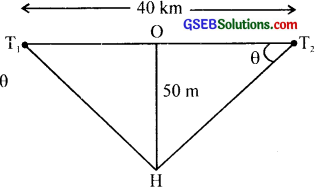d sin θ = nλ , λ = $$\frac {d sin θ}{n}$$
OT1 = OT2 = 20 km = 20 x 103m
For longest wavelength, n = l and sin θ ≈ θ ≈ tan θ
∴λ = $$\frac {d tan θ}{l}$$ = d tan θ = 50 x $$\frac{\mathrm{HO}}{\mathrm{OT}_{2}}$$
= $$\frac{50 \times 50}{20 \times 10^{3}}$$ = $$\frac {1}{8}$$ m =$$\frac {100}{8}$$cm = 12.5cm

Question 19.
A parallel beam of light of wavelength 500 nm falls on a narrow slit and the resulting diffraction pattern is observed on a screen 1 m away. It is observed that the first minimum is at a distance of 2.5 mm from the centre of the screen. Find the width of the slit.
Solution:
sin θ1 = $$\frac {λ}{a}$$
But sin θ1 = tan θ1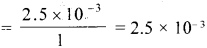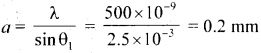Question 20.
(a) When a low flying aircraft passes overhead, we sometimes notice a slight shaking of the picture on our TV screen. Suggest a possible explanation.
(b) As you have learnt in the text, the principle of linear superposition of wave displacement is basic to understanding intensity distributions in diffraction and interference patterns. What is the justification of this principle?
Solution:
(a) Interference of the direct signal received by the antenna with the (weak) signal reflected by the passing aircraft.

(b) Superposition principle follows from the linear character of the solution of (differential) equation governing wave motion. If y1 and y2 are solutions of the wave equation. so is any linear combination of y1 and y2. When the amplitudes are large (e.g., high intensity laser beams) and non-linear effects are important. the situation is far more complicated.Question 21.
In deriving the single slit diffraction pattern, ¡t was stated that the intensity is zero at angles of $$\frac {nλ}{a}$$ Justify this by suitably dividing the slit to bring out the cancellation.
Solution:
Divide thc single slit into n smaller slits of width a’ = $$\frac {a}{n}$$ The angle θ = $$\frac {nλ}{a}$$ =$$\frac {λ}{a’}$$ Each of the smaller slits sends zero intensity in the direction O. The combination gives zero intensity as well.

### GSEB Class 12 Physics Wave Optics Additional Important Questions and Answers

Question 1.
What is the path difference between the two waves, when the’ crest of one wave falls on the crest of the other?
δ = n λ

Question 2.
What is the path difference between the two waves, when the crest of’ the wave fills on the trough of the other?
δ = (2n + 1)$$\frac {λ}{2}$$

Question 3.
What is constructive interference?
According to Huygens wave theory. a single source of light emits energy and spread out uniformly in all directions. But light energy emitted by two sources. due to superposition of waves gives rise to a resultant modification in light intensity. The modification of light intensity brought about by the superposition of two or more waves is called interference of light.

The law of conservation of energy holds good during interference. This phenomenon can be explained on the basis of the principle of superposition of waves. According to this principle, when to or more light waves interfere in a medium. the resultant dis placement at any point in the medium is the vector sum of the displacements due to individual waves. Consider two waves, with amplitude a1 and a2 [Fig. 10.7(a) and 10.7(b)]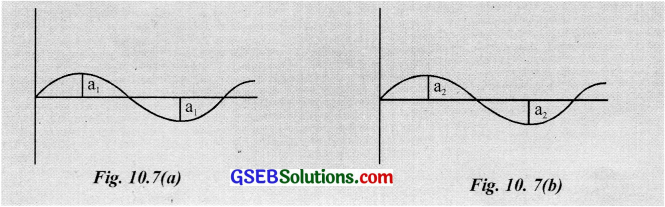If these two waves are superimposed, the following possibilities are observed.

(a) When crest of one falls on the crest of the other or trough of one falls on the trough of the other, in other ords if the aes superimpose in phase. then resultant amplitude (A) increases.
i.e..A = a1 + a2 or A = – (a1 + a2) …….(1)
This is called constructive interference.

(b) When crest of one falls on the trough of the other or vice-versa, in other words if the waves superimpose in opposite phase, then resultant amplitude (A) decreases.
i.e., A = a1 – a2 or A = a2 – a2 ………(2)
This is called destructive interference.If a1 = a2 then in first case (a). A = 2a and in the second case (b), A = 0
Intensity of a light wave is directly proportional to square of the amplitude. Fora single source. intensity I ∝ (amplitude)2. For constructive interference of two identical sources intensity is maximum
(Imax ) and Imax ∝A2 ………(3)
i.e.. Imax ∝ 4a2
i.e.. Imax is four times the intensity due to a single source.
For destructive interference. intensity is minimum and Imin ∝ A2 ……..(4)
i.e.. Imin = 0
If Imax and Imin are the intensities of maxima and minima produced by the two waves of amplitudes a1 and a2. then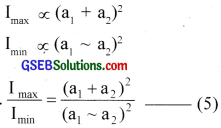In general, during superposition, there can be an arbitrary phase difference between the interfering waves. In such case, the resultant amplitude A is given by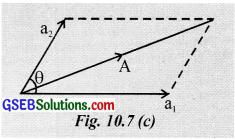A2 = a12 + a22 + 2a1a2 cos θ ……….(6)
The corresponding expression for intensity of resulting wave is
I = I1 + I2 + 2$$\sqrt{\mathrm{I}_{1} \cdot \mathrm{I}_{2}}$$ cos θ …….(7)
where I1 a12 and I2 ∝ a22

Condition for bright and dark fringes – Simple theory:
We can no develop a simple theory to show the general interference condition. S1 and S2 are two virtual sources obtained from a single monochromatic source S. Waves W1 and W2 coming respectively from S1 and S2 travel in an isotropic medium and interfere.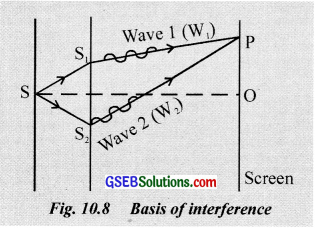Let P be a point where W and W, meet and produce interference pattern. The condition for maximum or minimum intensity of the pattern at P depends on the path difference between W1 and W2
Path of W1 = S1P
Path of W2 = S2P
∴Path difference between
W1 and W2 = S2P – S1P = δ ……..(1)
The condition for maximum or minimum intensity, as stated earlier, depends on phase difference also. Hence the relation between phase difference and path difference can be obtained as follows.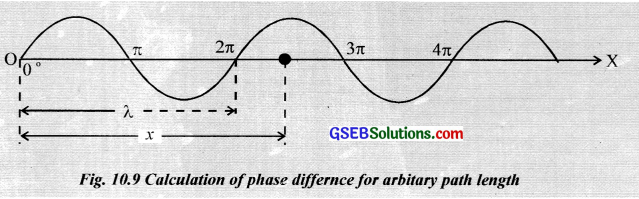Consider a monochromatic wave of wavelength λ, originating from o and propagating towards the positive direction of the X – axis. (sec. fig. 10.9) As the wave covers a distance equal to λ, the corresponding phase change of the particle at 0 is 2π
O is 2π. So for unit wavelength, the corresponding phase difference is $$\frac {2π}{λ}$$
If we take an arbitrary path length x, corresponding phase difference = $$\frac {2π}{λ}$$ × x
In the case of two intcrtring waves, W1 and W2 having a path difference δ the correspond ing phase difference is $$\frac {2π}{λ}$$ x δ If the phase difference is O, 2π, 4π, …….. 2nπ, where n is an
integer, the waves interfere in phase and maximum intensity is produced.
i.e., $$\frac {2π}{λ}$$ δ = 2nπ
δ = nλ or 2n $$\frac {λ}{2}$$ ………(2)i. e., path difference is an integral multiple of wavelength of light. Eq. (2) represents the condition for constructive ï interference (brightness).
If the phase difference is π, 3π, 5π ……. (2n ± 1) π, where n is an integer, the waves interkre in opposite phase and minimum intensity is produced.
i.e., $$\frac {2π}{λ}$$ δ = (2n±1)π
δ = (2n±l)$$\frac {λ}{2}$$ ………(3)
i.e., path difference is an odd multiple of half the wavelength of light. Eq.(3) represents the condition for destructive interference (darkness).

Coherent sources:
The two sources which emit continuous waves of same wavelength or frequencies, same amplitude and zero phase difference or constant phase difference are known as coherent sources. To maintain constant or zero phase difference, two sources should have exactly identical transitions which is never possible. Hence we cannot produce sustained interference pattern with two separate sources. We have to produce two sources from a single parent source. The two images of a single source can act as coherent sources. Because any’ phase change in real source will produce the
same phase change in the images also.

Conditions for sustained interference:

• By sustained interference pattern the positions of maximum and minimum intensity do not hange with time. To get sustained interference pattern the following conditions are to be satisfied.
• The two sources should continuously emit waves of same wavelength or frequency’.
• The amplitude of the two waves should be equal, if not there will not be total darkness at the position of dark fringe.
• The two waves should hase either zero phase difference or the phase difference should not change with time.
(Sources which satisfy’ all these three conditions are known as coherent sources).
• The two sources should he very close to each other.
• The sources should preferably be point source or narrow.
• The screen in which the interference pattern is obtained should be at comparatively large distance from the source.

Question 4.
In Young double slit experiment light waves are coherent. Why?
To obtain sustained interference.

Question 5.
If one of the slits is closed, what happens?
Only a white patch is obtained.

Question 6.
When both the slits are opened, we can see interference pattern. Why?
Due to the superposition of light waves coming from the two slits. Constructive and destructive interference are taking place.Question 7.
If two sources are used. what is the resultant amplitude of electric field and intensity of light for constructive interference?
2E and 4E2

Question 8.
For destructive interference, what is the value of resultant amplitude and intensity of light?
Zero. Zero

Question 9.
If one of the sills in the interference experiment is closed, what will be your observation?
Diffraction of light

Question 10.
Compare the intensifier of maxima and minima in terms of
a. hand width
b. Intensity ratio
a. Intensity (maximum or minimum) x Bandwidth
b. Intensity (maximum), Imax∝ (a1 + a2)2
Intensity (minimum). Iminimum∝minimum z (a1 – a1)2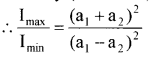Question 11.
Give an ail example of interference of light in our daily life situation.
Colours of thin him – Beautiful colours produced by a thin film of oil on the surface of the water.

Question 12.
Why does the picture on a TV screen shake slightly when a low-flying aircraft passes overhead?
It is due to the interference of the reflected signal from the aircraft and the direct signal received by the antenna.Question 13.
The diffraction pattern becomes invisible when ¡he slit is very wide. Give reason.
Slit width or size should be of the order of the wavelength of light to observe diffraction of light. Hence diffraction pattern is invisible if the slit is very wide.

Question 14.
The diffraction phenomenon is common in sound but not common in light. Why is it so?
Basic condition for diffraction to take place is that the size of the obstacle aperture should be of the order of the wavelength of waves used. For sound, the wavelength is large and we get obstacles of large size easily. hence diffraction is common in sound. In the case of light. wavelength is very small and small size obstacle is not available easily. Hence diffraction is not common in light.

Question 15.
(a) When we look through a muslin cloth, we can see coloured spectrum. How will you explain this?
(b) Observe the shadow of your hook when it is held a few centimeters above a table with a lamp several centimeters above the book. Why is the shadow of the book fuzzy at the edges?
(c) If you observe a distant street light between two fingers pinched together, you can visualise alternate bright and dark fringes. What is this due to? Explain.
(a) It is due to the diffraction of light through the fine fibres, which act as slits.
(b) When light falls on the edges of the book, the phenomenon of diffraction is also produced. But the pattern is not sharply identified. The cumulative effect is the formation of a blurred image.
(c) It is due to the diffraction of light. The slit so formed by the two fingers are fine whose size is comparable with the wavelength of light.

Question 16.
What longitudinal waves can be polarized?
OR
Light waves can be polarized while sound waves can’t Why?